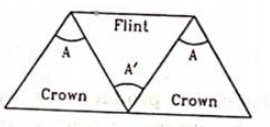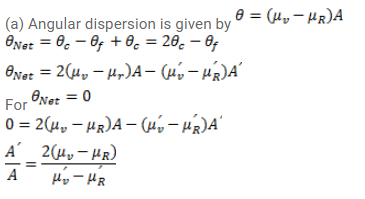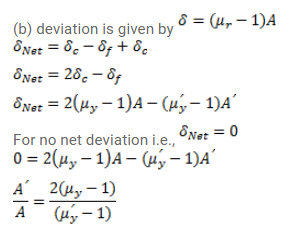# Three thin prisms are combined as shown in figure.Question:

Three thin prisms are combined as shown in figure. The refractive indices of the crown glass for red, yellow and violet rays are $\mu_{r}, \mu_{y_{\text {and }}} \mu_{v}$ respectively and those for the flint glass are $\mu_{r}^{\prime}, \mu_{y}^{\prime}$ and $\mu_{v}^{\prime}$ respectively. Find the ration $A^{\prime} / A$ for which

(a) there is no net angular dispersion and,

(b) there is no net deviation in the yellow ray.Solution: